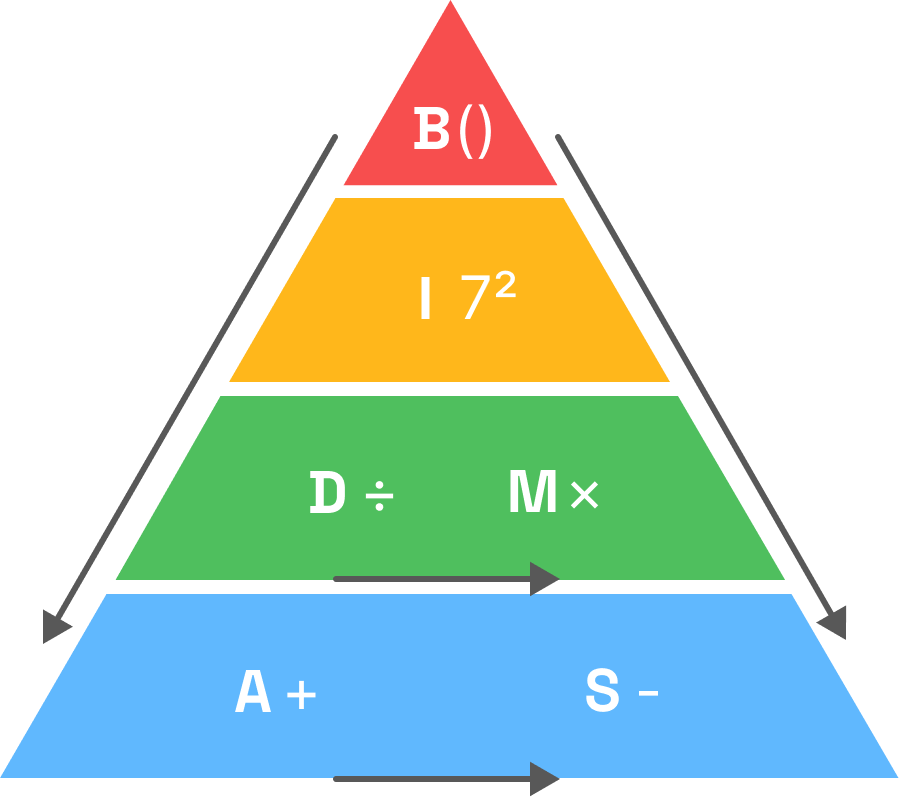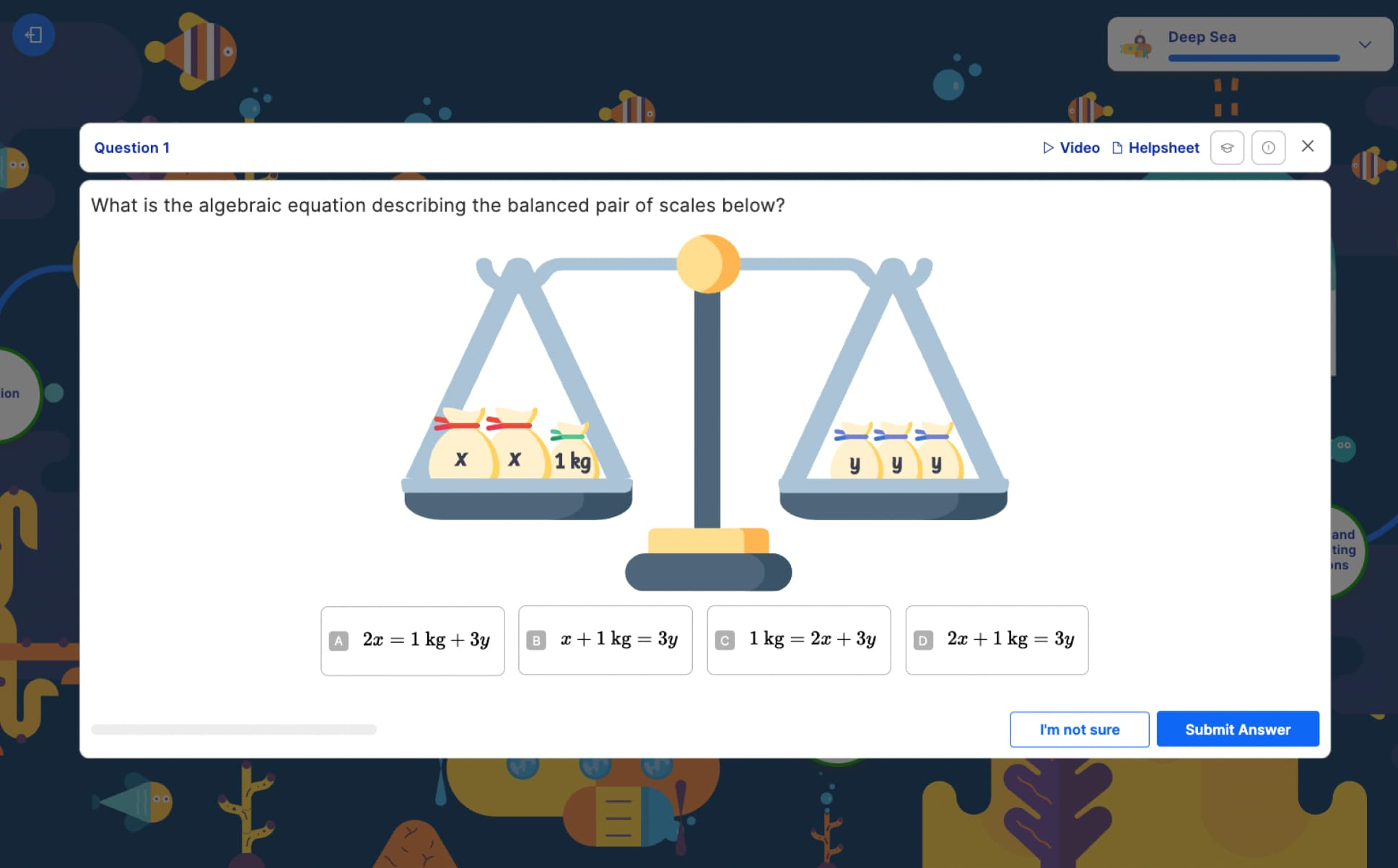Back to blog

# 11 plus challenging topics: maths

By Atom | Apr 6, 2023, 3:15 PMContents

In this series of free webinars, Atom’s Education Experts outline the areas of each 11 plus subject that our students struggle with the most, and share tips and techniques on how to prepare. Watch here, or keep reading for an overview:

Watch now

## The 11 plus maths curriculum

The 11 plus maths exam curriculum is mapped to the Key Stage 2 national curriculum. CEM and GL Assessments grammar school exams cover both Year 5 and Year 6 maths topics, whereas ISEB focuses on Year 5 topics.

Curriculum overview:

### Data

• Statistics: mode, median, range and mean

• Graphs: bar charts, pie charts, line graphs, scatter graphs, averages from graphs, tables, pictograms, venn diagrams

• Probability: basic probability, compound probability

### Number

• Place value: order and compare, Roman numerals, rounding numbers, negative numbers

• Operations: addition, subtraction, multiplication, division, mental maths, estimating, multiples, factors, prime numbers and factor trees, square and cube numbers, order of operations, worded problems

• Fractions: equivalent fractions, mixed numbers and improper fractions, order and compare fractions, adding and subtracting fractions, multiplying and dividing fractions

• Decimals: converting decimals and fractions, multiplying and dividing decimals, recurring decimals, order and compare decimals, adding and subtracting decimals

### Geometry

• Properties of shape: angles, polygons and circles, three-dimensional shapes, quadrilaterals, transformations

• Position and direction

### Measurement

• Space: area, volume, units of volumes and areas, surface area, length and perimeter

### Ratio and proportion

• Ratio: relative quantities, similar shapes and scale factors

• Proportion

• Percentages: order and compare percentages, percentage change, calculating percentages

### Algebra

• Expressions: forming expressions, algebraic notation, brackets in algebra, algebraic fractions

• Equations: substitution, solving, rearranging equations, inequalities, two unknowns

• Sequences: simple sequences, complex sequences, the nth term

About half to three-quarters of maths that is taught in Key Stage 2 (primary school Years 3–6) is within the number area (place value, fractions, operations and decimals).

Overall, the two skills that parents should treat as the highest priority for maths 11 plus preparation are number fluency and numerical reasoning.

• Number fluency: practice mental calculations, order of operations, and understanding fractions, decimals and percentages

• Numerical reasoning: practice understanding what is being asked in a question: translating worded problems into maths sums, and interpreting data and graphsA maths worded problem on Atom Nucleus

## The 3 maths topics students struggle with the most

Our data shows that there are three topics which Atom students who are preparing for the 11 plus find most difficult.

• Order of operations (number)

• Fractions topics (beyond basic fractions)

• Substitution and solving equations (algebra)

### Order of operations

Order of operations is the rule that tells us the sequence in which we should solve an expression with multiple operations. The acronym to remember this is BIDMAS (you may remember BODMAS from school):

• Brackets

• Indices (or Orders)

• Division and Multiplication

To avoid being tripped up by order of operations questions, it’s key to remember is that BIDMAS should be treated as a pyramid, not a list. Division and multiplication are one step, and addition and subtraction are one step.### Fractions

Working with fractions can be counterintuitive, so beyond the basics it is not usually possible to answer fractions on instinct alone. The fractions subtopics that children tend to find the hardest are mixed numbers and improper fractions, ordering and comparing fractions, adding/subtracting fractions, and multiplying/dividing fractions.

Here are the key points to remember to handle these questions confidently on the 11 plus:

• Fractions can only be added or subtracted when they have the same denominator

• Fractions can be greater than 1 (i.e. improper fractions such as 7/4, and mixed numbers such as 1 3/4)

• Ordering fractions can be counterintuitive. For example, 7/9 is greater than 7/10

• Multiplying by a fraction makes a number smaller, not bigger... and dividing by a fraction makes a number bigger!

### Algebra: substitution and solving equations

Many entrance exams don’t test algebra at all. When they do, it is often basic substitution questions, for example:

If x = 2 and y = 3, What is the value of 2x + 3y?

By the time the 11 plus comes around at the start of Year 6, your child is unlikely to have spent much, if any, time on algebra in the classroom yet. During Year 5, it’s a good idea to help your child gain a firm understanding of key algebra subtopics at home, followed by exploring learning beyond the foundations of algebra.

For Atom Nucleus subscribers, we recommend setting Custom Practices to begin introducing algebra from the middle of Year 5.An algebra practice question on Atom Nucleus

Make the most of the Lesson Library where your child can watch recordings of lessons on algebra and equations. Seeing the steps a teacher takes to solve a problem will help your child consolidate their learning.

Watch the webinar for free for more ways to support your child with these maths topics, plus exam techniques that will prepare them to take even the trickiest questions in their stride!## Free 11 plus practice paper bundle

• English, maths, verbal & non-verbal reasoning papers

• Mirror the content and format of standard GL grammar school exams

• Answer key and parents' guide